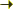Bilbao Crystallographic ServerIndex of a group-subgroup pair Help

## INDEX: Index of a group-subgroup pair

 Index of a group-subgroup pair
 The program INDEX calculates the index of a group subgroup pair. The index can be calculated in two different ways given: Option A The space group ITA numbers. The number of formula units per conventional unit cell. Option B The space group ITA numbers. The lattice parameters. The number of formula units per conventional unit cell Zc is simply related to the number Z of formula units per primitive unit cell via the so-called centring factor f, Z=Zc/f, with f=1 for primitive lattices, 2 for I-, C-, B-, A- centred, 3 for R-centred and 4 for F-centred lattice.
 Please, enter the sequential number of group as given in International Tables for Crystallography, Vol. A : Please, enter the sequential number of group as given in International Tables for Crystallography, Vol. A :

• Option A: Introduce the formula units (conventional) of the high and low symmetry structure.
 The formula units (conventional) on the high symmetry structure: The formula units (conventional) on the low symmetry structure:

• Option B: Introduce the lattice parameters of the high and low symmetry structure.
 The lattice parameters on the high symmetry structure: The lattice parameters on the low symmetry structure:

[ Bilbao Crystallographic Server Main Menu ]